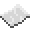# vector.cross

Given two vectors, self and object, this function will output their cross product. If the arguments do not follow Computercraft's vector style (a table with the contents: x, y, and z) the function could output undesired results.ExampleFind the cross product
Finds the cross product of two vectors
Code
```<nowiki>
local vectorA = vector.new(6, 7, -8)
local vectorB = vector.new(2, 3.2, 5)
local crossProduct = vector.cross(vectorA, vectorB)
print("The cross product of my two vectors is:", crossProduct)
</nowiki>
```
Output The cross product of my two vectors is: 60.6 -46 5.2ExampleBehind the scenes
An example of what the function looks like
Code
```<nowiki>
local vectorA = vector.new(6, 7, -8)
local vectorB = vector.new(2, 3.2, 5)
local crossProduct = vector.new(
(vectorA.y * vectorB.z - vectorA.z * vectorB.y),
(vectorA.z * vectorB.x - vectorA.x * vectorB.z),
(vectorA.x * vectorB.y - vectorA.y * vectorB.x))
print("The cross product of my two vectors is:", crossProduct)
</nowiki>
```
Output The cross product of my two vectors is: 60.6 -46 5.2

vector.cross
Function
Syntax
vector.cross(
• self : table
• object : table
)
Colon notation: self:cross(
• object : table
)

Returns table cross product
API vector
Source CC:Tweaked (source)